# Like Your Odds? Easily Compute Probability and Chance with Wolfram|Alpha

July 18, 2012 —Comments Off

A study of mathematical probability typically begins by considering a random situation with a low number of possible outcomes, like a coin flip or a toss of a die. Wolfram|Alpha has long been able to compute probabilities involving coins and dice.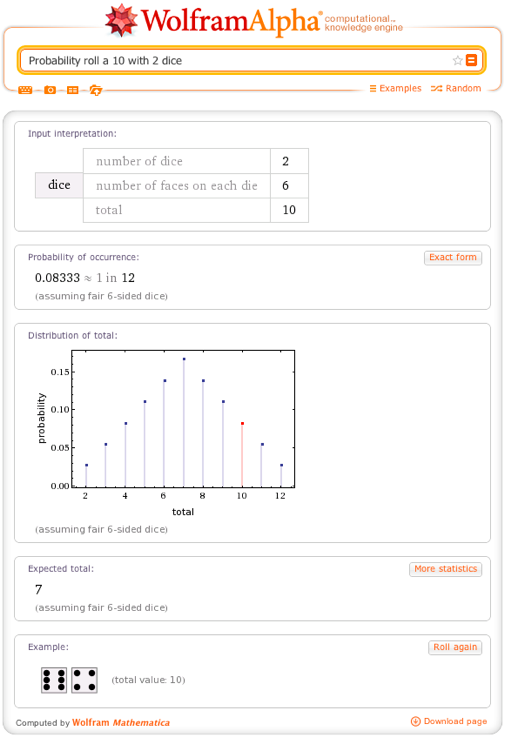These situations are modeled mathematically by identifying all of the possible outcomes (the sample space) and assigning a probability to each in some reasonable way. This assignment of probabilities is called a distribution. The possible totals that can be shown with a pair of dice are {2,3,4,5,6,7,8,9,10,11,12}, and the probability associated with each is the height of the corresponding bar in the “Distribution of total” pod.

The bar heights in this pod are derived mathematically from assumptions about the dice, but what makes them a good model is that they are a good reflection of the fraction of times the corresponding outcome is observed when one rolls a pair of dice many times, as is easily verified in Mathematica. The plot below shows the fraction of times each total comes up in one million simulated tosses of two dice, and it looks very much like the theoretical distribution given by Wolfram|Alpha above.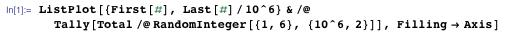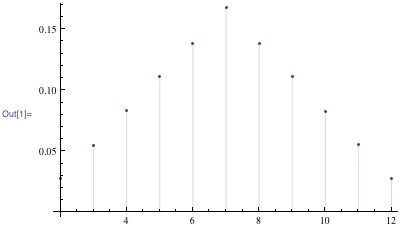Of course, if the dice were loaded somehow so that each face was not equally likely to land face up, this distribution might no longer be a good model (hence the fine print below the plot in Wolfram|Alpha). There are many ways to distribute probability—we need only ensure that the probabilities are not negative and add up to 1. This allows us to invent a great variety of probability models to address different kinds of problems.

Some probability distributions that have proved especially useful have been named, and Wolfram|Alpha knows many of them. For example, the binomial distribution distributes probability among the possible counts of heads in n flips of a coin that is weighted so that the probability of a single flip landing heads is p. Like most named distributions, the binomial distribution is really a family of distributions that share many common features but vary somewhat as one changes the “parameters” n and p. The function that performs the distributing of probability (i.e. gives the heights of the bars) is called a probability density function.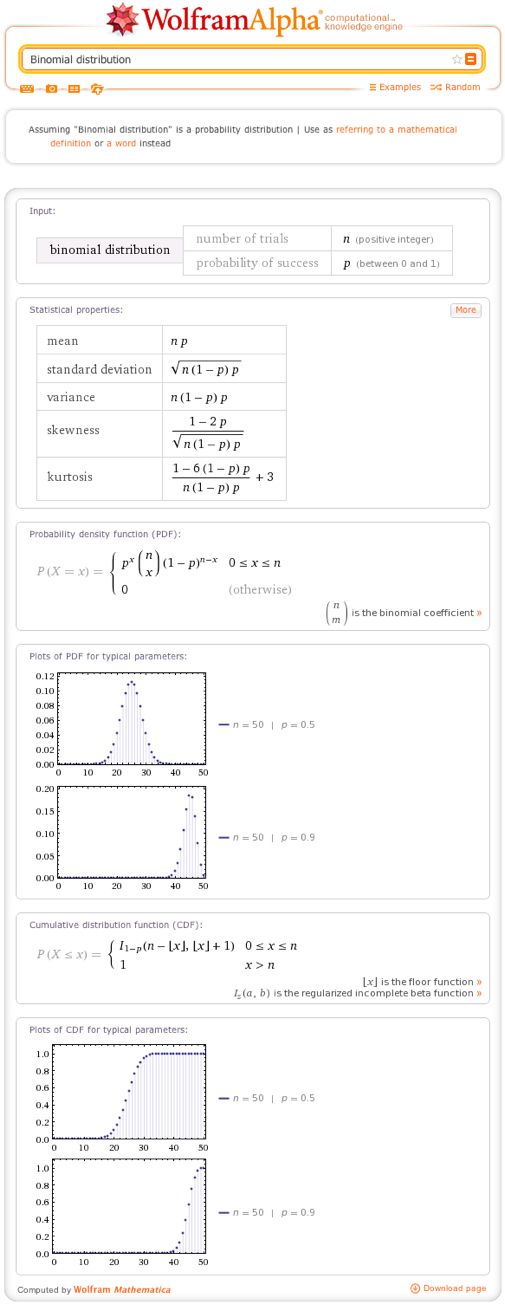With p = .7, the distribution is skewed to the right, because high numbers of heads are more likely: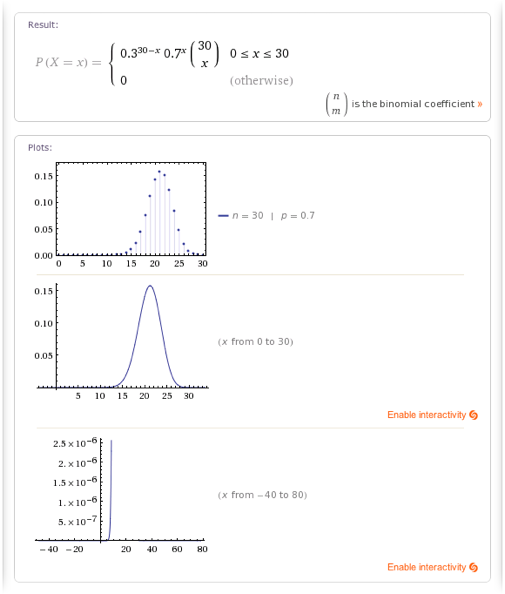With p = .5, heads and tails are equally likely on any given flip, so the distribution is perfectly symmetric: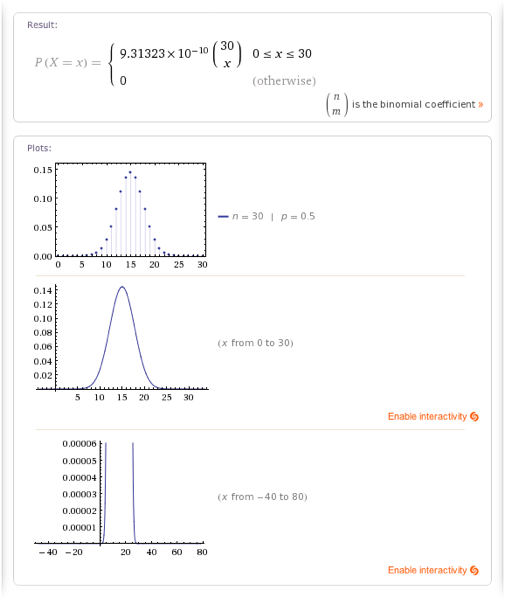Wolfram|Alpha can compute probabilities from distributions as well. In probability parlance, the number of heads in 30 flips is called a random variable. We use some variable name to represent it in forming probability queries.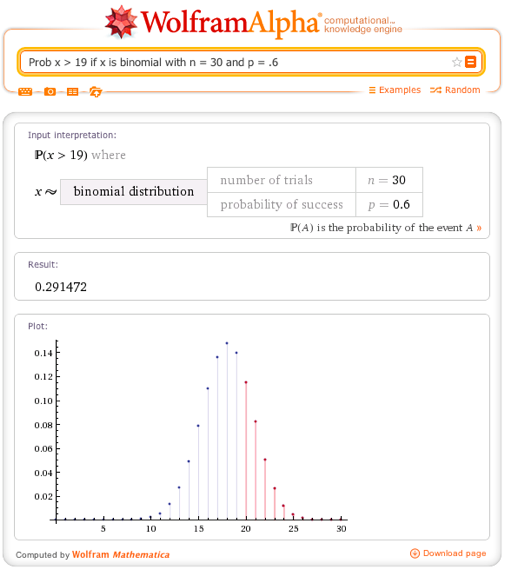In terms of coins, the query above asks for the probability of observing more than 19 heads in 30 flips of a coin weighted so that the probability of a single flip landing heads is 0.6. Notice that geometrically, this probability is calculated by summing the lengths of the red segments in the plot—the probability that there are 20 heads plus the probability that there are 21 heads and so on.

Distributions like these take a line segment of unit length and divide it into pieces—the pieces are the bars shown in the distribution. A shortcoming of this is that we can’t distribute probability across a continuous interval of values in this manner, only across a discrete set of values. To accomplish the former, we imagine taking a unit of area and stretching it across a continuous interval. The function that accomplishes this continues to be called a probability density function.

Here’s an example. The blue area in the plot pod is 1, and the bell-shaped curve gives one way of distributing this area across the entire real line. Probabilities are assigned not so much to individual values as to ranges of values, and the probability of a range of values is the fraction of blue area that lies over that range. Distributions like this are called continuous distributions, while distributions like the binomial distribution are called discrete distributions.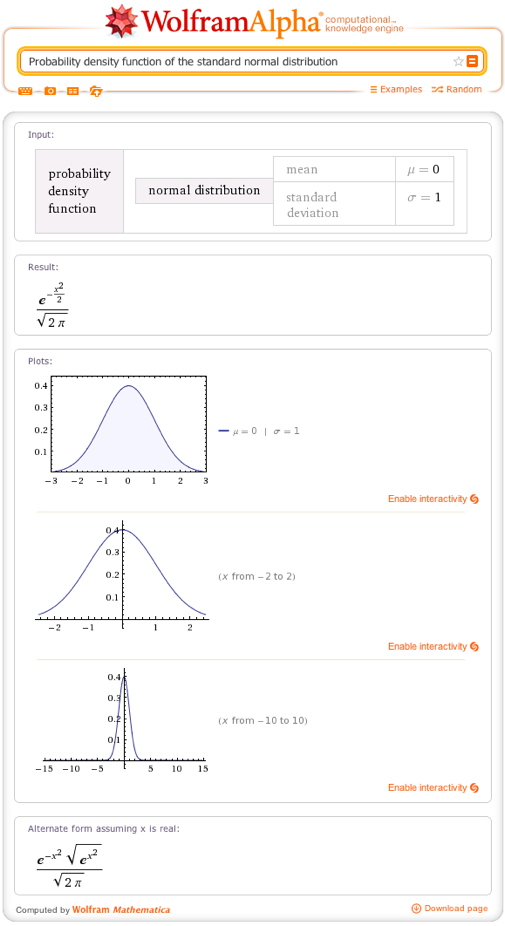Computing exact probabilities for continuous distributions generally requires calculus. Here’s an example in Wolfram|Alpha: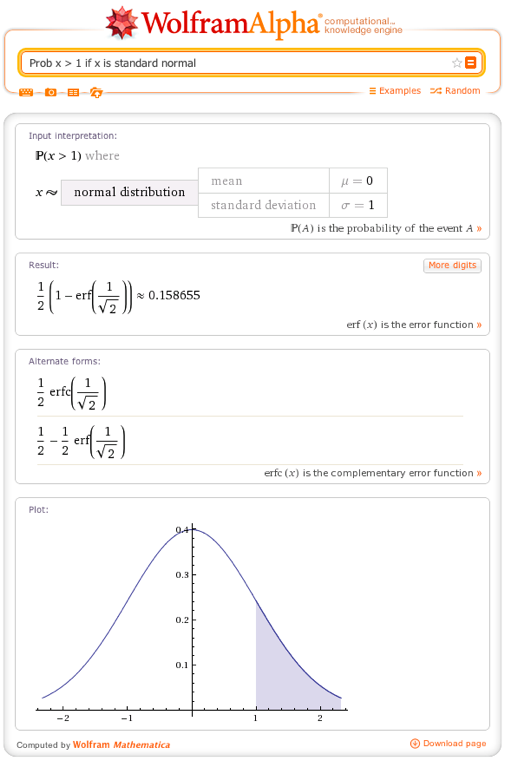As in the discrete case, there are many families of continuous distributions that have been named. The most famous of these is undoubtedly the normal distribution, which uses a bell-shaped curve whose center and narrowness are adjusted by the parameters μ and σ, respectively.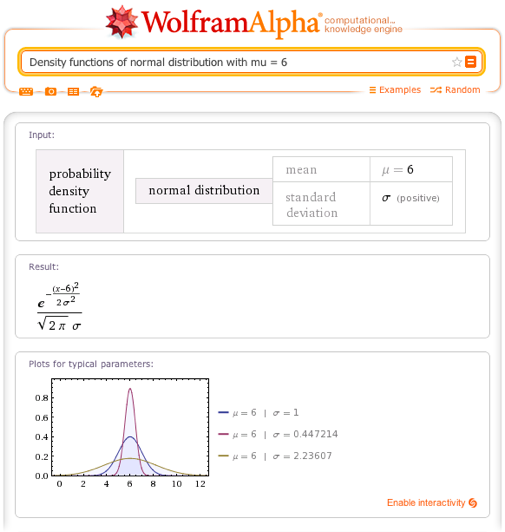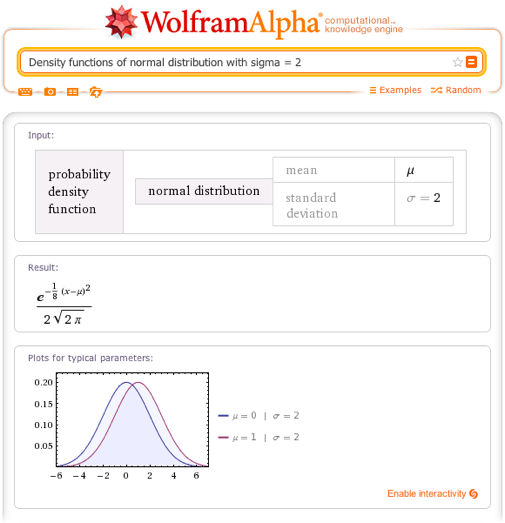Wolfram|Alpha’s ability to easily compute and visualize probabilities from distributions is liberating. Such computations have traditionally either been avoided (in academic settings) or have required highly technical calculations or significant programming ability. Here are a couple examples using distribution families other than the normal distribution: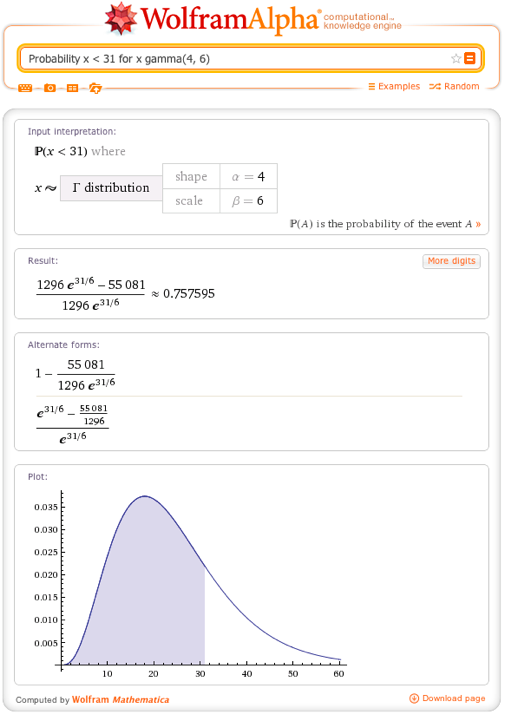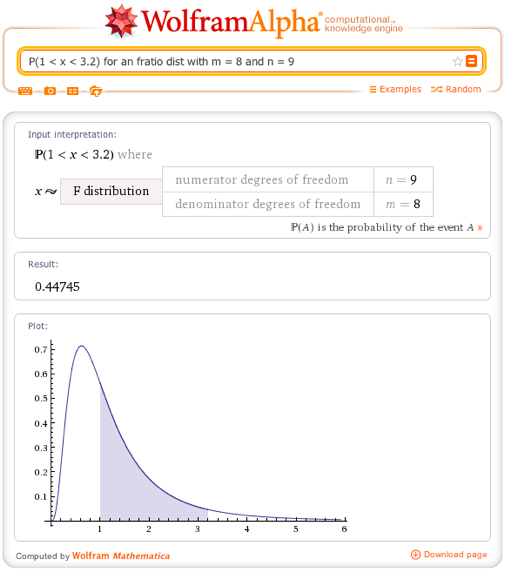Of course, our efforts to make Wolfram|Alpha the best possible tool for computing and understanding probability are ongoing, and we look forward to getting feedback from you and bringing you more and better probability functionality in the future.

Wow!

Posted by Jeff July 18, 2012 at 2:10 pm

I have problems to calculate probability in the form of equality (x = 3) for discrete distribution like binomial or poisson, for example:
P(x=3) for x~binomial(10; 0.3)
The similar tasks with any inequalities works OK, for example:
P(x>=3) for x~binomial(10; 0.3)
or
P(2<x<4) for x~poisson(3)
I think it may be an error because probability in the form of equality is a legal task for discrete random variables. Also input interpretation is anomalous, it begins with First[{}]=… instead of x=…

Of course the equality x = 3 may be written in the form of inequality 2 < x < 4 which works OK. But why so complicated?

Is is an error in Wolfram Alpha or I am wrong?
(I use Wolfram Alpha in my statistics lessons.)
Thanks
Vicq

Posted by Vaclav Friedrich aka Vicq March 13, 2014 at 1:44 pm

Thanks! You are correct–we’re working on getting this fixed within Wolfram|Alpha.

Posted by The Wolfram|Alpha Team March 19, 2014 at 3:45 pm

number of different dice that can be made having the property that the numbers on opposite faces are consecutive natural numbers among 1 2 3 4 5 and 6

Posted by Daks April 22, 2014 at 10:26 am

I have a data set where I have calculated the probability of 2025 independent outcomes–trying to determine the overall probability of all possible combinations of groups of 5. Any thoughts? A bit daunting given there are 282,356,400,741,030 total possible combinations to consider…

Posted by Wes July 7, 2015 at 4:33 pm

This i simple. Add up all of the dots on 2 dice (12) and start adding with one… 1+1… 1+2

It is small enough it will take a considerably small amnt of time.

Posted by Pigeon Fever September 8, 2015 at 5:22 pm